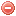# Description

The Baumgartner index is a fire danger index developed by Baumgartner et al. (1967) in Bavaria (Germany), based on the concept that fire danger is mainly conditioned by fuel dryness which, in turn, depends on evapotranspiration. Baumgartner et al. (1967) thus designed an index consisting of the difference between potential evapotranspiration - after the Penman (1948) formulation - and precipitation.

The calculation of the Baumgartner index requires daily precipitation [mm] and the potential evapotranspiration after Penman (1948) as input variables.

Although it was designed there, Langholz & Schmidtmayer (1993) found the Baumgartner index relatively unsuitable for predicting fire danger in Bavaria, above all in spring when dead litter flammability depends less on the precipitation of the last five days than on current or short-term drought conditions (cf. section "Formula").

# Formula

The Baumgartner index $$BI$$ is calculated as follows (Baumgartner et al. 1967):

$BI_t=\displaystyle \sum_{i=1}^{5}{\bigg(PET_{t-i}-P_{t-i}\bigg)}$

where $$PET$$ is the sum of the potential evapotranspiration [mm] according to Penman (1948) and $$P$$ the sum of precipitation [mm] during the five days preceding the day for which the index has to be calculated.

The Baumgartner index is supposed to be calculated on a daily basis. The data for calculating the potential evapotranspiration have to be recorded at 2 pm.

The calculation of the Baumgartner index should to be started after snowmelt in late winter/spring. No particular starting value is stipulated.

# Interpretation

Baumgartner et al. (1967) proposed a fire danger classification according to the output values of their index:

Month Fire danger class
low slight moderate high very high
March < 5 5 - 3 3 - 9 9 - 15 > 15
April < 3 3 - 8 8 - 16 16 - 27 > 27
May < 3 3 - 16 16 - 25 25 - 35 > 35
June < 12 12 - 24 24 - 32 32 - 41 > 41
July < 12 12 - 24 24 - 31 31 - 40 > 40
August < 8 8 - 20 20 - 28 28 - 37 > 37
September < 6 6 - 18 18 - 26 26 - 35 > 35

Unfortunately, no fire danger classification was provided for winter months.

Original publication:
Baumgartner et al. (1967)

Other publication:
Penman (1948)

### Bibliography SearchNo results!

No results were found. Are you sure you searched for a tag?

### Symbols

 Variable Description Unit $$T$$ air temperature °C $$T_{dew}$$ dew point temperature °C $$H$$ air humidity % $$P$$ rainfall mm $$U$$ windspeed m/s $$w$$ days since last rain (or rain above threshold) d $$rr$$ days with consecutive rain d $$\Delta t$$ time increment d $$\Delta{e}$$ vapor pressure deficit kPa $$e_s$$ saturation vapor pressure kPa $$e_a$$ actual vapor pressure kPa $$p_{atm}$$ atmospheric pressure kPa $$PET$$ potential evapotranspiration mm/d $$r$$ soil water reserve mm $$r_s$$ surface water reserve mm $$EMC$$ equilibrium moisture content % $$DF$$ drought factor - $$N$$ daylight hours hr $$D$$ weighted 24-hr average moisture condition hr $$\omega$$ sunset hour angle rad $$\delta$$ solar declination rad $$\varphi$$ latitude rad $$Cc$$ cloud cover Okta $$J$$ day of the year (1..365/366) - $$I$$ heat index - $$R_n$$ net radiation MJ⋅m-2⋅d-1 $$R_a$$ daily extraterrestrial radiation MJ⋅m-2⋅d-1 $$R_s$$ solar radiation MJ⋅m-2⋅d-1 $$R_{so}$$ clear-sky solar radiation MJ⋅m-2⋅d-1 $$R_{ns}$$ net shortwave radiation MJ⋅m-2⋅d-1 $$R_{nl}$$ net longwave radiation MJ⋅m-2⋅d-1 $$\lambda$$ latent heat of vaporization MJ/kg $$z$$ elevation m a.s.l. $$d_r$$ inverse relative distance Earth-Sun - $$\alpha$$ albedo or canopy reflection coefficient - $$\Delta$$ slope of the saturation vapor pressure curve kPa/°C $$Cc$$ cloud cover eights $$ROS$$ rate of spread m/h $$RSF$$ rate of spread factor - $$WF$$ wind factor - $$WRF$$ water reserve factor - $$FH$$ false relative humidity - $$FAF$$ fuel availability factor - $$PC$$ phenological coefficient -

 Suffix Description $$-$$ mean / daily value $$_{max}$$ maximum value $$_{min}$$ minimum value $$_{12}$$ value at 12:00 $$_{13}$$ value at 13:00 $$_{15}$$ value at 15:00 $$_{m}$$ montly value $$_{y}$$ yearly value $$_{f/a}$$ value at fuel-atmosphere interface $$_{dur}$$ duration $$_{soil}$$ value at soil level

 Constant Description $$e$$ Euler's number $$\gamma$$ psychrometric constant $$G_{SC}$$ solar constant $$\sigma$$ Stefan-Bolzmann constant